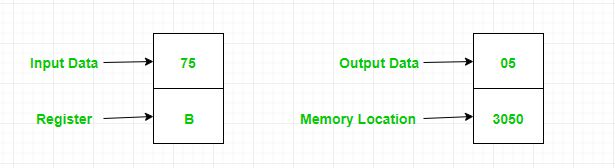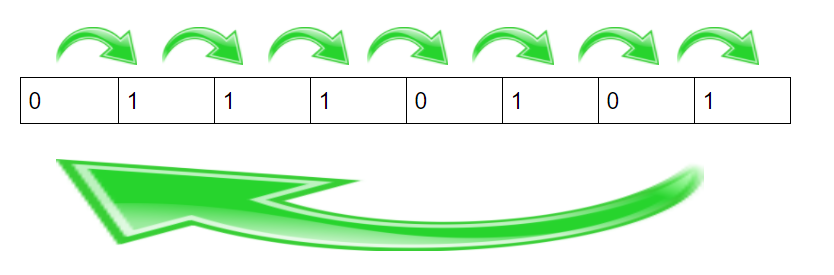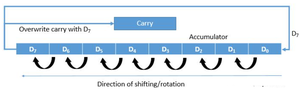GFG App
Open AppBrowser
Continue

# 8085 program to count the number of ones in contents of register B

Problem: Write an assembly language program to count the number of ones in the contents of register B and store the result at memory location 3050.

Example:Algorithm:

• Convert the decimal number in Accumulator to its binary equivalent.
• Rotate the digits of the binary number right without carry.
• Apply a loop till the count is not zero to change the values of the D register and count.
• Copy the value of the D register to the accumulator and store the result.

Program:

Explanation:

1. MVI B 75 moves 75 decimal numbers into the B register
2. MVI C 08 moves 08 decimal number into C register, which is taken as counter as the number is of 8 bytes
3. MVI D 00 moves 00 number into d register
4. MOV A, B moves the contents of B register into A (accumulator) register
5. RRC rotates the contents of A (which is 75 with binary equivalent 01110101) right6. JNC 200C jumps to 200C address and performs the instructions written there if the carry flag is not zero
7. INR D increases the value of the D register by adding one to its contents
8. DCR C decreases the value of the C register by subtracting one from its contents
9. JNZ 2007 jumps to the 2007 address and performs the instructions written there if the zero flags are not zero
10. MOV A, D moves the contents of the B register into the A register
11. STA 3050 store the contents of A at 3050 memory location
12. HLT stops execution

Approach : 2 [Using Rotate Instruction RLC] :

Here algorithm will be the same as above but here we are moving contents of accumulator bits to 1-bit left and then checking carry flag values and updating the count register accordingly.

If Carry Flag = 1 , then Count = Count + 1

Otherwise: Rotate Accumulator Left again without carrying and repeat the above procedure.Both Different Instructions do the same task and concept of achieving end-goal is one and the same. Only Rotating Style is Different but these 2 methods can do the same task for us.

### Advantages of counting ones using this program:

• It is a simple and efficient way to count the number of ones in the contents of register B using only a few instructions.

• It is a low-level operation that provides a deep understanding of the internal workings of the 8085 microprocessor.

• It can be easily adapted for use in other programs and applications that require counting the number of ones in binary data.

### Disadvantages of counting ones using this program:

• It requires specialized knowledge of assembly language programming and the 8085 microprocessor architecture.

• It may not be efficient for larger sets of data, as it involves looping through each bit of the register and checking for a carry.

• It may not be practical for use in high-level programming languages or applications where speed and efficiency are critical.
My Personal Notes arrow_drop_up